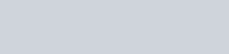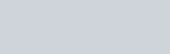# Summation and Product Notation

## Introduction

In this article we are going to talk about the summation and product notation, what they mean and how to perform the calculations. At the end we will see how to program summation and product notation in Java or C# language.

## What is summation and product notation?

Summation and product are ways of defining mathematical operations that consist of sequences of sums and products respectively.

Imagine that we have to add or multiply all the numbers starting at 1 and ending at 1000, but also multiply each of them by 3.

Using symbology we can write long operations like that in short. In addition the properties can help us to simplify the expression and to calculate the results.

## Sigma Notation

In the next video you can see the concept of the sigma notation and an excercise solved using programming techniques in Unity.

##### Implement sigma notation in programming🟢

The summation is symbolized by the Greek letter Sigma in capital letters.

Below the summation symbol the index variable is indicated and from which value it starts. At the top of the symbol is the last value to be taken by the index.

To the right of the symbol is the expression that determines all the terms of the summation, each term arises from replacing the index in the expression from the first to the last value.

In equation 1 we see on the left side the expression of the summation and on the right side the developed operation.

(1)## How to program a Summation?

To implement the summation in some programming language is relatively simple, we must define a variable to accumulate the sum that must be initialized in 0 (neutral element for the sum). We have to take into account which numerical set the summands belong to, this will depend on the type of variable to choose.

We define an integer variable for the end of the summation.

We make a for loop that goes from the beginning of the summation to the end and inside the loop we define the operation to make.

In figure 1 we see the implementation of the sum of equation 1 together with the printed result in console.Fig. 1: Implementation of the summation of equation 1 in Java, Netbeans IDE.

## Symbol of Product notación

The product notation is symbolised by the letter Pi in capital letters.

Under the producer symbol is indicated the index variable and from which value it starts. In the upper part of the symbol is indicated the last value that the index will take.

To the right of the symbol is the expression that determines all the terms of the producer, each term arises from replacing the index in the expression from the first to the last value.

In equation 2 we see on the left side the expression of the producer and on the right side the developed operation.

(2)## Implement the product notation in programming

We must define a variable to accumulate the product that must be initialized in 1 (neutral element of the product). We also analyze the type of numbers we are multiplying to choose the type of the accumulation variable.

We define a whole variable for the end of the producer.

We make a for loop that goes from the beginning of the production to the end and inside the loop we define the operation to be performed.

In figure 2 we see the implementation of the producer of equation 2 together with the printed result in console.Fig. 2: Implementation of the equation 2 producer in Java, Netbeans IDE.